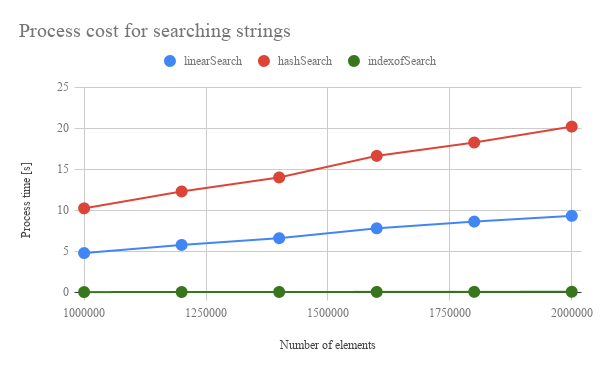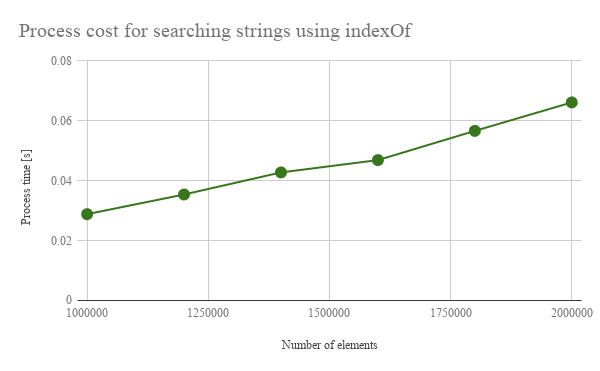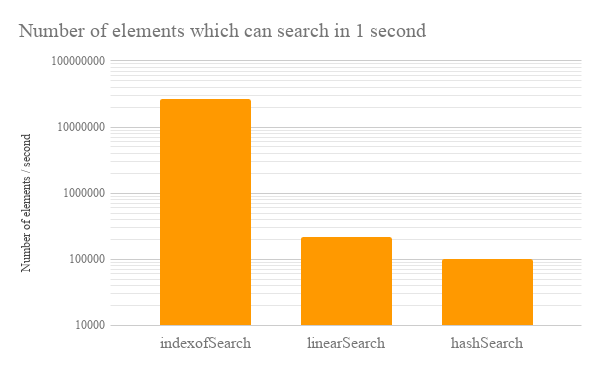Gists

Kanshi Tanaike

# Introduction

Please be careful! This result can be only used for Google Apps Script.

There are a limit executing time for Google Apps Script (GAS). That is 6 minutes.1 So users always have to pay attention to reducing the process cost of the scripts. Especially, it is very important to know the process cost for the array processing, because the array processing is often used for spreadsheet and Google APIs. Recently, I have reported about the process cost of the loop for the array processing.2 Also I have reported “Improved Algorithms for Summation of Array Elements” as a method for reducing the process cost.3 From these reports, it has found that GAS shows much different process cost from other languages. So it is important to investigate the process cost for various scenes. In this report, the process cost of “searching strings in an array” for the array processing using GAS has been investigated.

Here, as a sample, it supposes the situation that it searches 3 strings from one dimensional array. When users use the process for this situation using GAS, the following 3 methods can be considered.

1. Search using linear search by for loop
2. Search using hash by creating an object
3. Search using indexOf() 4

From the report of loop cost, 2 it has already found that the loop by map and filter is the fastest at GAS. 2 But in this report, “for loop” which is the general loop was used.

As the result, it was found that the cost of search by indexOf() was the lowest of all methods. Namely, it was the fastest of all. 2nd and last one were the search by for loop and the search by the hash, respectively. In the case of the search by hash, it was fount that although the cost of search by the hash from the object is very low, the cost for creating the object to search the hash was the highest of all. By this, the search by the hash became the lowest rank. If the object for searching has already been created, the cost of search by the hash will be the lowest of all.

# Experimental procedure

In the experiment, the sample scripts for GAS were used. 1 dimensional array including 3 strings for searching was used as a source array. The 3 string values were put to the top, center and end of the array in order to measure the same condition. In this case, it uses all of elements in the array. 3 methods as mentioned above were used for searching the strings from the array. As the measurements of process cost, those 3 strings were searched from the source array by changing the number of elements of the array, and the process cost which is the search time was measured. In each search, the index of array which has the searched string was retrieved. The cost for creating array was not included. Only the cost for searching was measured. By the way, at GAS, the processing time is not stable as you know. So the average value for more than 250 times measurements was used for each data point which is shown by figures. At this time, the fluctuation of the average values was less than 1 %. I worry that each detailed-data point at my environment might be different from that at other user’s environment. But I think that the trend of this result can be used.

# ResultsFig 1. Number of elements vs. processing time (process cost) for searching 3 strings. The solid lines with the color of red, blue and green mean the result from the search using hash, the linear search and the search using indexOf, respectively.Fig 2. Each method vs. processing efficiency time (process cost) for searching strings. Here, the chart of search using indexOf shown in Fig. 1 was magnified.

Figure 1 shows the number of elements vs. processing time (process cost) for searching 3 strings. At figure 2, the chart of search using indexOf shown in Fig. 1 was magnified. From Fig. 1, it was found that the search using indexOf is the fastest of all. The second is the linear search. The last is the search using hash. It is considered that the reason of this order is as follows.

1. From the sample script, both the linear search and the search using hash process all elements in the array using the for loop. On the other hand, the search using indexOf doesn’t process all elements using the for loop. The index of the element with the found string is directly retrieved using indexOf. When the string is found, the elements in the array are removed from the start index to the index of element with the found string. By this, the array length becomes small. This flow is repeated until to find all strings for searching. So it is considered that the search cost of indexOf which doesn’t process all elements using the for loop became the lowest of all. This may indicate that the scan for indexOf() is different from it for the general for loop.
2. In the case of the search by hash, it was found that although the cost of search by the hash from the object is very low, the cost for creating the object to search the hash was the highest of all. So it is considered that the search by hash became the lowest rank. If the object for searching has already been created, the cost of search by the hash will be the lowest of all.
3. The linear search processes all elements using the for loop as same as the search using hash. At the linear search, the elements are scanned and the searched string is retrieved using if. On the other hand, at the search using hash, the object is created to search while using if. After the object was created, the strings are searched. From the result of order of cost, it is considered that the cost for creating an object is higher than that of push(). So it is considered that the linear search became the second.Fig 3. Number of elements which can be searched per unit time. Vertical axis is the logger scale. Horizontal axis is each method. These were calculated using the slopes of each method in Figs 1 and 2.

Figure 3 shows the number of elements which can be searched per unit time. The vertical axis and horizontal axis are the logger scale and each method, respectively. These values were calculated using the slopes of each method in Figs 1 and 2. From Fig. 3, it was found that the search using indexOf can reduce the process cost of more than 99 % from the linear search and the search using hash.

# Summary

In this report, the process cost of “searching strings in an array” for the array processing by 3 methods using GAS was investigated. As the result, it was found the following important features for GAS.

• Process cost of search by indexOf() was the lowest of all methods.
• 2nd and last one were the search by for loop and the search by the hash, respectively.
• About the search by hash, although the cost of search by the hash from the object is very low, the cost for creating the object to search the hash was the highest of all. By this, the search by hash became the lowest rank. If the object for searching has already been created, the cost of search by the hash will be the lowest of all.
• Search using indexOf can reduce the process cost of more than 99 % from the linear search and the search using hash.
• From these results, it is considered that the scan for indexOf() may be different from the general for loop.

As a note, I have to describe that this is the result for Google Apps Script. For other languages, this result might be difference. And also, the process cost of this report might be modified by future update of Google.

## Appendix

### Scripts

#### Creating sample arrays

The function for creating an array is as follows. “searchText” is put to the center, top and end of the array. In this report, the script searches the index of array elements with “searchText” from the array including 3 “searchText”.

function createSampleArray(max, searchText) {
var r = Array.apply(null, new Array(max)).map(function(_, i) {return "val" + ('0000000000' + i).slice(-8)});
// Put the search value to center of the array.
Array.prototype.splice.apply(r, [Math.floor(r.length / 2), 0].concat([searchText]));
// Put the search value to top of the array.
r.unshift(searchText);
// Put the search value to end of the array.
r.push(searchText);
return r;
}


The array becomes like below.

[
"searchText",
"val00000000",
"val00000001",
"val00000002",
"val00000003",
,
,
,
"searchText",
,
,
,
"val00000097",
"val00000098",
"val00000099",
"val00000100",
"searchText"
]


#### Searching values

// Linear search
function linearSearch(array, searchText) {
var result = [];
for (var i = 0; i < array.length; i++) {
if (array[i] == searchText) {
result.push(i);
}
}
return result;
}

// Search using hash
function hashSearch(array, searchText) {
var hash = {};
for (var i = 0; i < array.length; i++) {
if (hash[array[i]]) {
hash[array[i]] = hash[array[i]].concat(i);
} else {
hash[array[i]] = [i];
}
}
return hash[searchText];
}

// Search using indexOf
function indexofSearch(array, searchText) {
var result = [];
var base = 0;
var len = array.length;
for (var i = 0; i < len; i++) {
var c = array.indexOf(searchText);
if (c > -1) {
base += c;
result.push(i + base);
array.splice(0, c + 1);
} else {
break;
}
}
return result;
}